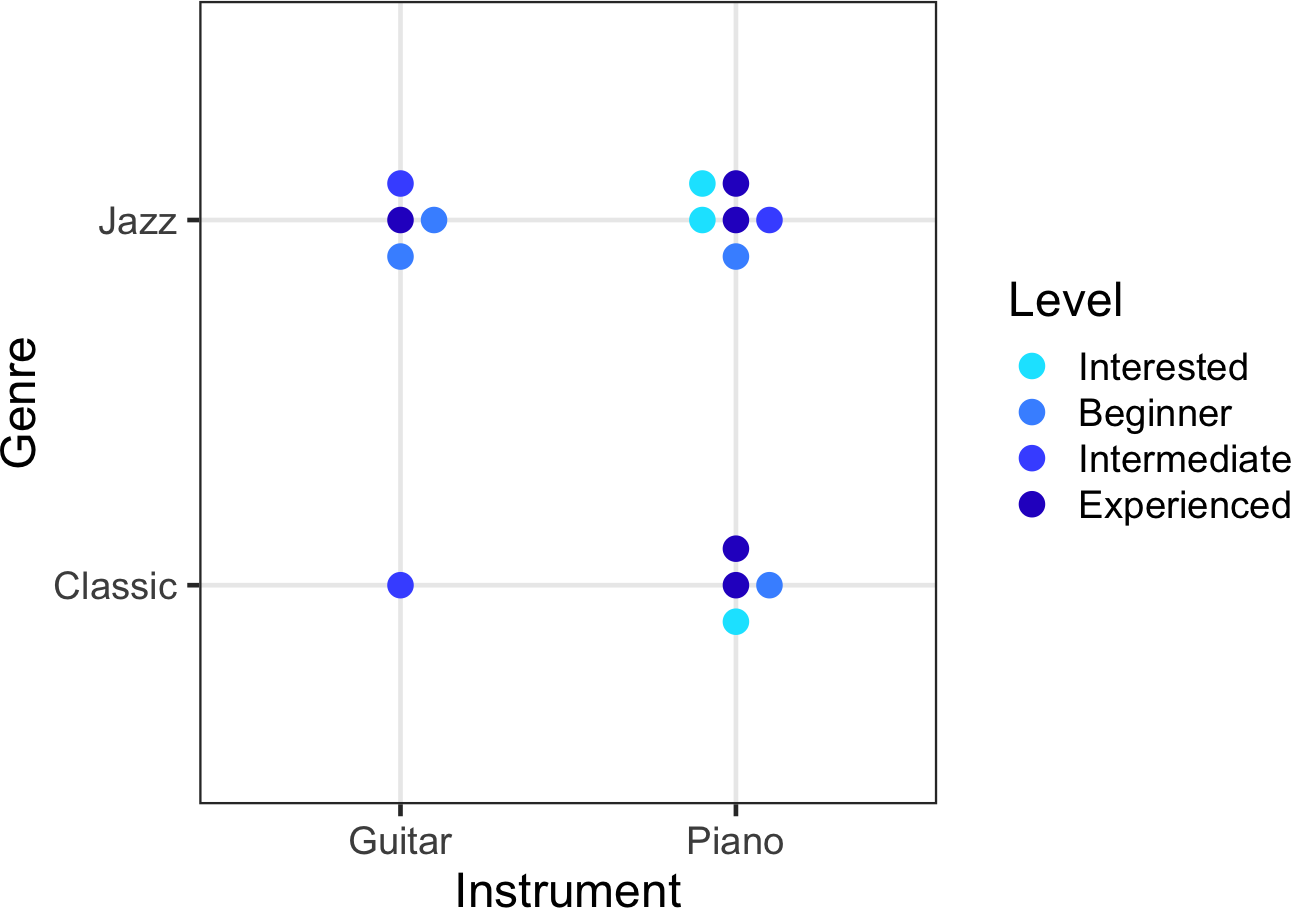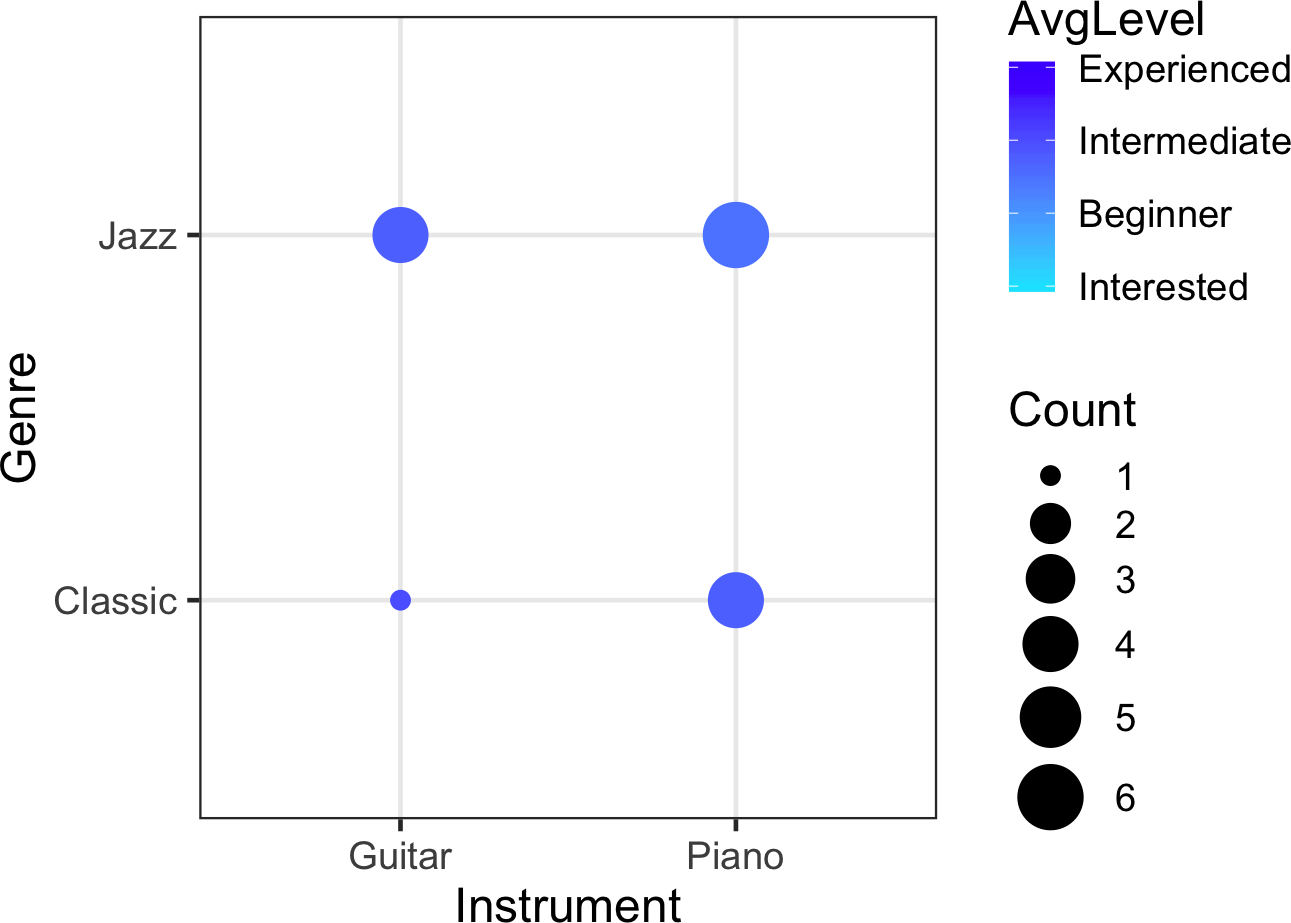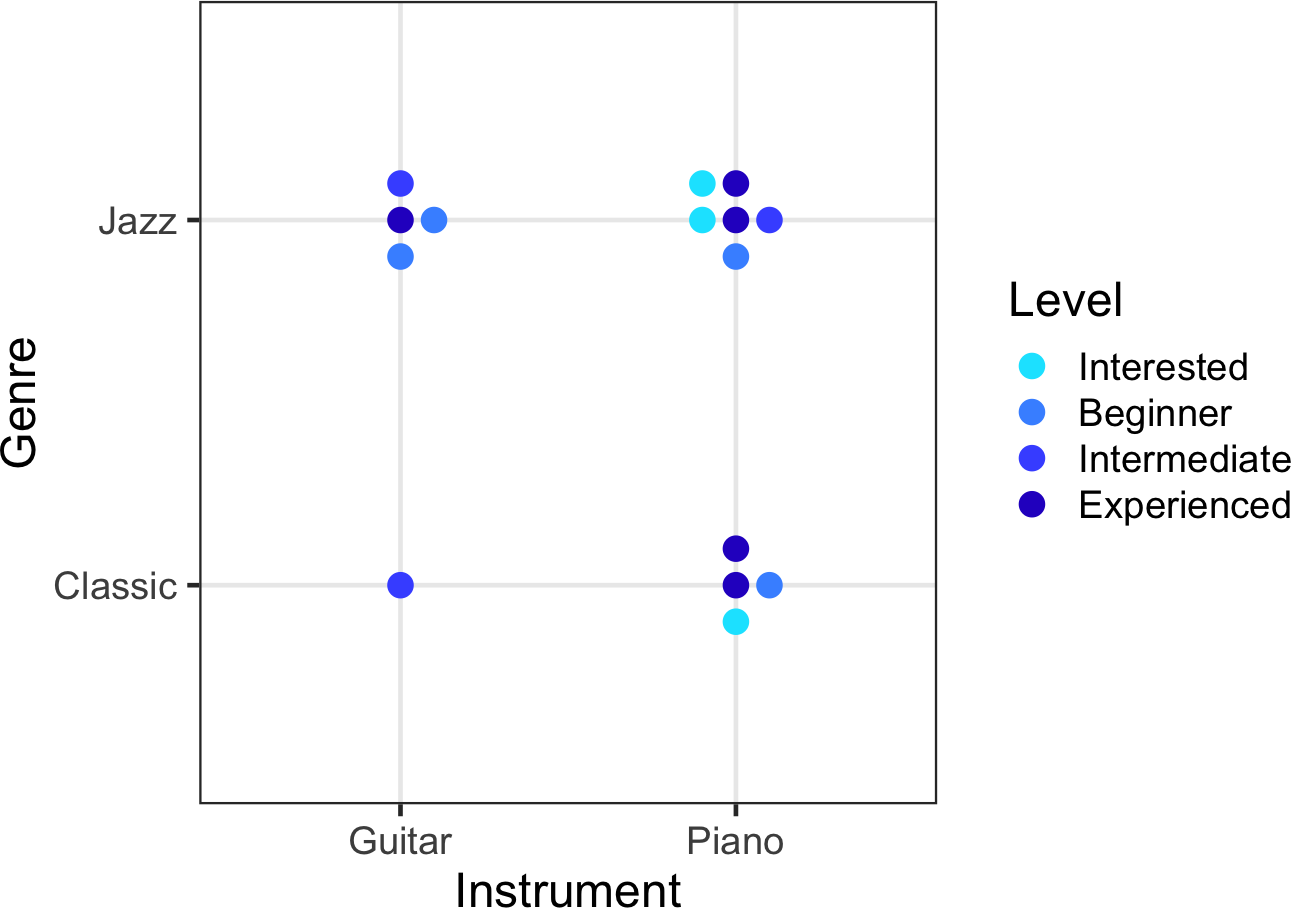# 1 Introduction

Welcome to this vignette!

## 1.1 Loading the package

You can load the package with this command

``````require(ggplot2)
require(ggBubbles)``````

## 1.2 In 15 seconds

The package introduces `position_surround()` for ggplot2.

Parameter is `offset` which controls the offsets for position corrections (default is 0.1).

`position_surround()` can be used in many ggplot2 functions like `geom_point` or `geom_text`:Figure 1: Example of a MiniBubble plot

# 2 Bubbleplot vs Minibubble plot

Here we demonstrate the advantage of MiniBubble plots compared to traditional Bubbleplot in certain usecases with discrete data.

Please not that in this vignette we will use dplyr and tibble from tidyverse.

``````require(dplyr)
require(tibble)``````

## 2.1 Example data

First, we load a small example data

``data(MusicianInterestsSmall)``

which contains data from musicians about their experience in differente music genres they have with their music instruments.

``head(MusicianInterestsSmall)``

Table 1: First rows of MusicianInterestsSmall
Instrument Genre Level
Piano Jazz Experienced
Piano Jazz Experienced
Piano Jazz Intermediate
Piano Jazz Beginner
Piano Jazz Interested
Piano Jazz Interested

## 2.2 Traditional Bubble plot

The traditional bubble plot is able to portrait the amount of guitarrists or pianists able to play jazz or classical music by size and display the average experience level by colour coding.

``````ggplot(data = MusicianInterestsSmall %>%
group_by(Instrument, Genre) %>%
summarize(Count = n(), AvgLevel = mean(as.integer(Level))),
aes(x = Instrument, y = Genre, size = Count, col = AvgLevel)) +
geom_point() + theme_bw(base_size = 18) +
scale_colour_gradientn(
colours  = rev(topo.colors(2)),
na.value = "transparent",
breaks   = as.integer(MusicianInterestsSmall\$Level) %>%
unique %>% sort,
labels   = levels(MusicianInterestsSmall\$Level),
limits   = c(as.integer(MusicianInterestsSmall\$Level) %>% min,
as.integer(MusicianInterestsSmall\$Level) %>% max)) +
scale_size_continuous(range = c(3, 11)) ``````Figure 2: Traditional Bubble Plot

From a data visualisation point of view, it is debateable how good point sizes are to display counts. However, in general we can agree that averages often hide a lot of useful information.

## 2.3 MiniBubble Plot

The MiniBubble plot allows to show each musician and their corresponding skill level individually:

``````ggplot(data = MusicianInterestsSmall,
aes(x = Instrument,
y = Genre,
col = Level)) +
geom_point(position = position_surround(), size = 4) +
scale_colour_manual(values = c("#00e5ff",
"#4694ff",
"#465aff",
"#2c00c9")) +
theme_bw(base_size = 18)``````Figure 3: Example of a MiniBubbleplot with position_surround

This is done by the `position_surround()` function passed to the `position` argument of `geom_point`. Note, that only exact overlaps will be dodged. The points will surround the center in layers which will be filled clockwise.

Since each individual data point is shown seperately, you can also use `shape` and `fill` to show further features, as long the plot will not be overloaded with information.

Also, you can use `geom_text(position = position_surround())` to overlay the points with text, or make the text appear in shiny when hovering.

MiniBubbleplot allows to show more features in a bubble plot.

# 3 Offset parameter

The offset of the dodged points can be handed as parameters to `position_surround()`.

``````ggplot(data = MusicianInterestsSmall,
aes(x = Instrument,
y = Genre,
col = Level)) +
geom_point(position = position_surround(offset = .2), size = 4) +
scale_colour_manual(values = c("#00e5ff",
"#4694ff",
"#465aff",
"#2c00c9")) +
theme_bw(base_size = 18)``````